# Calculating moving average in Excel

In this short tutorial, you will learn how to quickly calculate a simple moving average in Excel, what functions to use to get moving average for the last N days, weeks, months or years, and how to add a moving average trendline to an Excel chart.

In a couple of recent articles, we have taken a close look at calculating average in Excel. If you've been following our blog, you already know how to calculate a normal average and what functions to use to find weighted average. In today's tutorial, we will discuss two basic techniques to calculate moving average in Excel.

## What is moving average?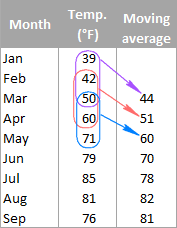Generally speaking, moving average (also referred to as rolling average, running average or moving mean) can be defined as a series of averages for different subsets of the same data set.

It is frequently used in statistics, seasonally-adjusted economic and weather forecasting to understand underlying trends. In stock trading, moving average is an indicator that shows the average value of a security over a given period of time. In business, it's a common practice to calculate a moving average of sales for the last 3 months to determine the recent trend.

For example, the moving average of three-month temperatures can be calculated by taking the average of temperatures from January to March, then the average of temperatures from February to April, then of March to May, and so on.

There exist different types of moving average such as simple (also known as arithmetic), exponential, variable, triangular, and weighted. In this tutorial, we will be looking into the most commonly used simple moving average.

## Calculating simple moving average in Excel

Overall, there are two ways to get a simple moving average in Excel - by using formulas and trendline options. The following examples demonstrate both techniques.

### Example 1. Calculate moving average for a certain time period

A simple moving average can be calculated in no time with the AVERAGE function. Supposing you have a list of average monthly temperatures in column B, and you want to find a moving average for 3 months (as shown in the image above).

Write a usual AVERAGE formula for the first 3 values and input it in the row corresponding to the 3rd value from the top (cell C4 in this example), and then copy the formula down to other cells in the column:

`=AVERAGE(B2:B4)`

You can fix the column with an absolute reference (like \$B2) if you want to, but be sure to use relative row references (without the \$ sign) so that the formula adjusts properly for other cells.

Remembering that an average is computed by adding up values and then dividing the sum by the number of values to be averaged, you can verify the result by using the SUM formula:

`=SUM(B2:B4)/3`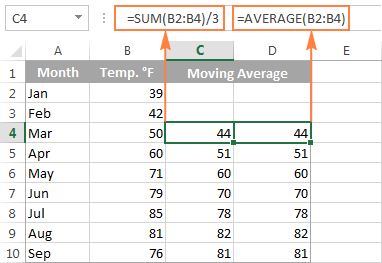### Example 2. Get moving average for a the last N days / weeks / months/ years in a column

Supposing you have a list of data, e.g. sale figures or stock quotes, and you want to know the average of the last 3 months at any point of time. For this, you need a formula that will recalculate the average as soon as you enter a value for the next month. What Excel function is capable of doing this? The good old AVERAGE in combination with OFFSET and COUNT.

=AVERAGE(OFFSET(first cell, COUNT(entire range)-N,0,N,1))

Where N is the number of the last days / weeks / months/ years to include in the average.

Not sure how to use this moving average formula in your Excel worksheets? The following example will make things clearer.

Assuming that the values to average are in column B beginning in row 2, the formula would be as follows:

`=AVERAGE(OFFSET(B2,COUNT(B2:B100)-3,0,3,1))`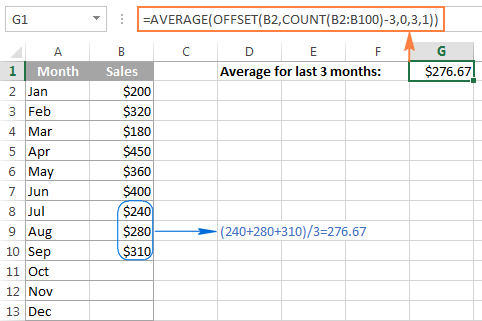And now, let's try to understand what this Excel moving average formula is actually doing.

• The COUNT function COUNT(B2:B100) counts how many values are already entered in column B. We start counting in B2 because row 1 is the column header.
• The OFFSET function takes cell B2 (the 1st argument) as the starting point, and offsets the count (the value returned by the COUNT function) by moving 3 rows up (-3 in the 2nd argument). As the result, it returns the sum of values in a range consisting of 3 rows (3 in the 4th argument) and 1 column (1 in the last argument), which is the latest 3 months that we want.
• Finally, the returned sum is passed to the AVERAGE function to calculate the moving average.
Tip. If you are working with continuously updatable worksheets where new rows are likely to be added in the future, be sure to supply a sufficient number of rows to the COUNT function to accommodate potential new entries. It's not a problem if you include more rows than actually needed as long as you have the first cell right, the COUNT function will discard all empty rows anyway.

As you probably noticed, the table in this example contains data for only 12 months, and yet the range B2:B100 is supplied to COUNT, just to be on the save side :)

### Example 3. Get moving average for the last N values in a row

If you want to calculate a moving average for the last N days, months, years, etc. in the same row, you can adjust the Offset formula in this way:

=AVERAGE(OFFSET(first cell,0,COUNT(range)-N,1,N,))

Supposing B2 is the first number in the row, and you want to include the last 3 numbers in the average, the formula takes the following shape:

`=AVERAGE(OFFSET(B2,0,COUNT(B2:N2)-3,1,3))`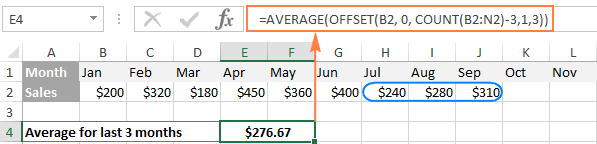## Creating an Excel moving average chart

If you have already created a chart for your data, adding a moving average trendline for that chart is a matter of seconds. For this, we are going to use Excel Trendline feature and the detailed steps follow below.

For this example, I've created a 2-D column chart (Insert tab > Charts group) for our sales data: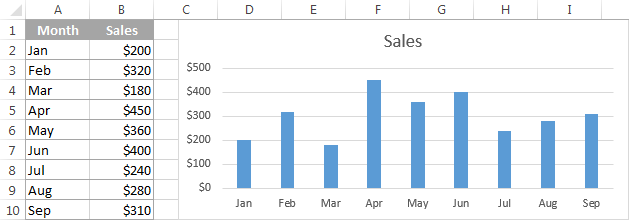And now, we want to "visualize" the moving average for 3 months.

1. In Excel 2013, select the chart, go to the Design tab > Chart Layouts group, and click Add Chart Element > Trendline > More Trendline Options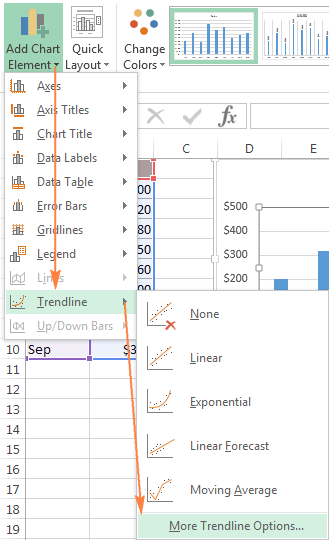In Excel 2010 and Excel 2007, go to Layout > Trendline > More Trendline Options.

Tip. If you do not need to specify the details such as the moving average interval or names, you can click Design > Add Chart Element > Trendline > Moving Average for the immediate result.
2. The Format Trendline pane will open on the right-hand side of your worksheet in Excel 2013, and the corresponding dialog box will pop up in Excel 2010 and 2007.

On the Format Trendline pane, you click the Trendline Options icon, select the Moving Average option and specify the moving average interval in the Period box: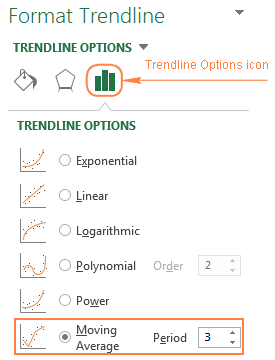3. Close the Trendline pane and you will find the moving average trendline added to your chart: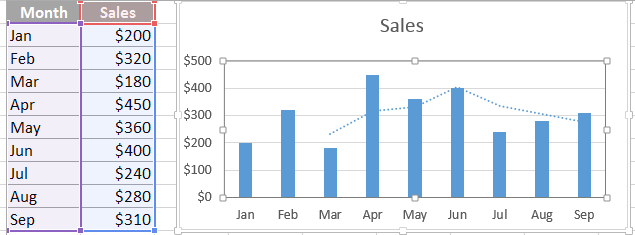To refine your chat, you can switch to the Fill & Line or Effects tab on the Format Trendline pane and play with different options such as line type, color, width, etc.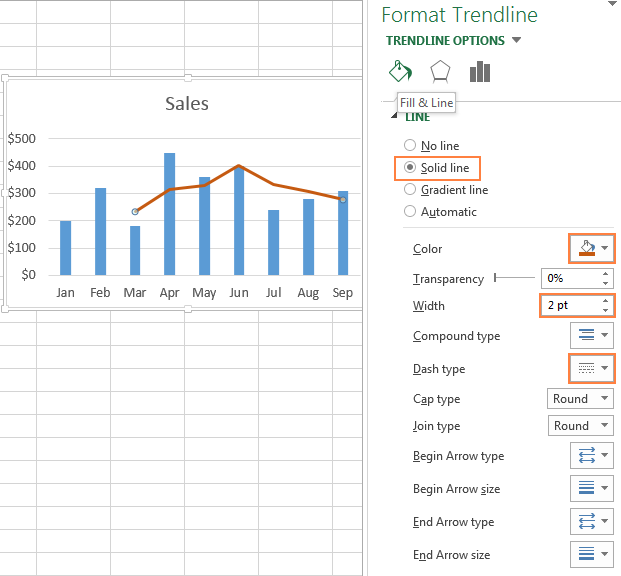For powerful data analysis, you may want to add a few moving average trendlines with different time intervals to see how the trend evolves. The following screenshot shows the 2-month (green) and 3-month (brick red) moving average trendlines: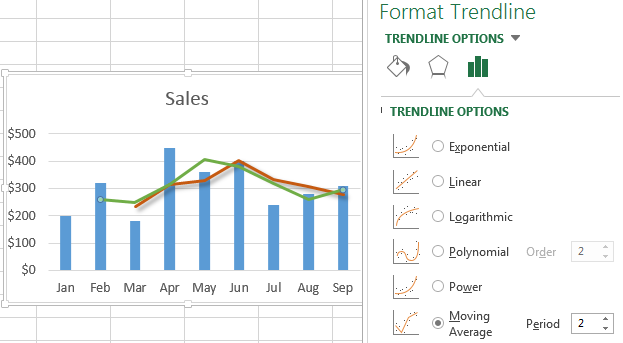Well, that's all about calculating moving average in Excel. The sample worksheet with the moving average formulas and trendline is available for download - Moving Average spreadsheet. I thank you for reading and look forward to seeing you next week!

### 71 responses to "Calculating moving average in Excel"

1.Kay says:

Hi,
I have read through several different articles looking for an answer to my problem, got lots of great tips but nothing defiant that helps me now.
Could you please help me on this? I’m trying to create a formula that adds the total amount for a particular supplier that is more than 30 days & in another cell a formula that is more than 60 days, etc…
I have the dates of invoices in column A, supplier’s names in column B and Total value in column C.
Any help would be appreciated, have spent days on this & to no avail. Thanks

•Svetlana Cheusheva (Ablebits.com Team) says:

Hi Kay,

I think you can use the SUMIFS function. For example, to find the total for supplier "John" for orders made more than 30 days ago, you can use the following formula:

=SUMIFS(C2:C100, B2:B100, "john", A2:A100,"< "&TODAY()-30)

For the detailed explanation of SUMIFS' arguments and more formula examples, please see How to use Excel SUMIFS and SUMIF with multiple criteria.

2.Yogi says:

Hi,

I need your help on this please. I have "column A" with dates and "column B" with values which has both negative and positive values in it. i want to calculate "average" for a particular date from "columb B". Can you please help me on how to calculate.

3.Yogi says:

Hi,

I need your help on this please. I have "column A" with dates and "column B" with values which has both negative and positive values in it. i want to calculate "average" for a particular date from "columb B". Can you please help me on how to calculate average only for the positive values from "column B".

•Maria Azbel (Ablebits.com Team) says:

Hello, Yogi,

Please try one of these formulas:
=AVERAGEIF(B:B,"= 42386",A:A) or
=AVERAGEIF(B:B,B3,A:A)

In these formulas please replace 42386 or B3 with the needed parameter.

4.Kofi says:

Trying to calculate moving averages for working capital budget. ie moving averages for inventory, trade debtors and trade creditors.
Any Help?

5.Noman says:

I have face a problem. I want to create a sheet in excel, where 1st day(1st column contain daily score, 2nd column contains TTL score,and 3rd column contains average) as same 4th-6th column 2nd day, as same 7th-9th column. please replay me by mail,who are able to help to create it.

•Maria Azbel (Ablebits.com Team) says:

Hello, Noman,

Please send us a small sample table with your data in Excel and include the result you need to get to support@ablebits.com. We'll see if we can help.

6.charlie says:

Hi, I have 4 weekly figures so can work out the running total, but how do I work out an average weekly total when I'm adding new figures every week which will need to be included in the weekly figure. ie this week I need the average weekly total of 4 figures but next week it will be for 5 figures.
Thanks

7.Ami says:

Hi,
I am trying to get a moving average for the 7 previous days, the day itself and then the following 6 days (fortnight avg for a week either side of the day).
Can you help?
thanks

8.Arqui says:

Hi,
I am trying to calculate the average of a given project for the last 4 weeks, how can I do this since the cell will not always be next to each other.

9.SA says:

Hi,
I have some daily data, with column A having dates and column B having values. I want to calculate the average of the values occurring between two specific dates, for instance between 1/1/2016 to 2/15/2016. How would I be able to do that?

10.nikhil says:

Hi,
I have 1000 numbers starting from 1 to 1000. i want average for every 3 numbers. say from 1 to 3 it should be 2. now my next number will be 4 to 6 then 7 to 10 and so on up to 1000 numbers.

If i use average function then it will do average for 1 to 3 then 2 to 4 then 3 to 5. it is not meeting my requirement.

•atul says:

Hi nikhil,

You can use average (indirect ("b"&rows (b\$1\$:b1)*3-2&":"&"b"&rows(b \$1\$:b1)*3))

To calculate average for column b for every 3 sets of numbers

11.atul says:

Hi nikhil,

12.atul says:

You can use average (indirect ("b"&rows (b\$1\$:b1)*3-2&":"&"b"&rows(b \$1\$:b1)*3))

To calculate average for column b for every 3 sets of numbers

13.Todd says:

I have 2 rows, 1st row 2015 sales Jan -Dec (per month),
2nd row 2016 sales Jan - Dec (per month). I want to do a running average for 2016 vs 2015 (2016/2015=%). example; 2016 Jan vs 2015 Jan = %, as i add the next months sales i would need 2016 Jan + Feb vs 2015 Jan + Feb = % , and so on. Is this possible?

14.Rishabh says:

Hi .. I really liked the way it has been explained and was able to do in in a minute.. I also liked your website and will follow it regularly..

I was working with a e excel file where i required to make a moving average of last 2 months data which were in different column i.e(D3:G2)

Can you please let me know what modification is required in the formula so that i can make it work to calculate the same..

Rishabh

•Svetlana Cheusheva (Ablebits.com Team) says:

Hi Rishabh,

Thank you for your feedback. Assuming that you need a moving average for the last 2 numbers in the same row, you can adjust the formula in this way:

=AVERAGE(OFFSET(D3, 0, COUNT(D3:Z3)-2,1,2))

Where D3 is the first number in the row, and 2 is the number of the last N months to be included in the average.

15.Rishabh says:

Wow.. Thank you so much Svetlana :)

16.Rekha says:

Hi,

similar to CAGR which calculates compound annual growth rate in values, is there a formula for calculating the same growth between months. viz, Jan to June of the same year

17.vishakha says:

Hii,

I wanted to track facebook likes on my page on daily basis. Like i want to compare today's likes with previous day's average, whether its hike or less.

18.SHAROOQ KHAN says:

sir / mam , i want to calculate ratio of employees on the basis of "INTIME"
thank you.

19.Paulette says:

Your example 3 above (Get moving average for the last N values in a row) worked perfectly for me if the whole row contains numbers. I'm doing this for my golf league where we use a 4 week rolling average. Sometimes the golfers are absent so instead of a score, I will put "ABS" (text) in the cell. I still want the formula to look for the last 4 scores and not count the "ABS" either in the numerator or in the denominator. How do I modify the formula to accomplish this?

•Ralph says:

Yes, I did notice if cells were empty the calculations were incorrect. In my situation I am tracking over 52 weeks. Even if the last 52 weeks contained data, the calculation was incorrect if any cell prior to the 52 weeks was blank.

20.Archie Mendrez says:

I'm trying to create a formula to get the moving average for 3 period, appreciate if you can help pls.

Date Product Price
10/1/2016 A 1.00
10/1/2016 B 5.00
10/1/2016 C 10.00
10/2/2016 A 1.50
10/2/2016 B 6.00
10/2/2016 C 11.00
10/3/2016 A 2.00
10/3/2016 B 15.00
10/3/2016 C 20.00
10/4/2016 A 4.00
10/4/2016 B 20.00
10/4/2016 C 40.00
10/5/2016 A 0.50
10/5/2016 B 3.00
10/5/2016 C 5.00
10/6/2016 A 1.00
10/6/2016 B 5.00
10/6/2016 C 10.00
10/7/2016 A 0.50
10/7/2016 B 4.00
10/7/2016 C 20.00

21.Archie Mendrez says:

any body please can help to set excl formula to get the daily moving ave of product A, B, and C for the given sample details

22.Sandy Bodell says:

I want to do an hourly moving average of data that has a different number of observations for each hour (2 to 6 observations).

Can you help?

23.James Brown says:

Hi, I am impressed with the vast knowledge and the concise and effective instruction you provide. I too have a query which I hope you can lend your talent with a solution as well.
I have a column A of 50 (weekly) interval dates. I have a column B next to it with planned production average by week to complete target of 700 widgets (700/50). In the next column I sum my weekly increments to date (100 for example) and recalculate my remaining qty forecast avg per remaining weeks (ex 700-100/30).
I would like to replot weekly a graph starting with the current week (not the beginning x axis date of the chart), with the summed amount (100) so that my starting point is the current week plus the remaining avg/week (20), and end the linear graph at end of week 30 and y point of 700.
The variables of identifying the correct cell date in column A and ending at goal 700 with an automatic update from today's date, is confounding me.
Could you help please with a formula? (I've been trying =IF logic with =Today and just not solving it.)
Thank you

24.Johnny Muller says:

Please help with the correct formula to calculate the sum of hours entered on a moving 7 day period. For example. I need to know how much overtime is worked by an individual over a rolling 7 day period calculated from the beginning of the year to the end of the year. The total amount of hrs worked must update for the 7 rolling days as I enter the overtime hours in on a daily basis
Thank you

•Harvey says:

I am looking for something very similar! I am looking for the last 6 months! I'm so stumped!

25.Harvey says:

Hello Everyone,

Is there a way to get a sum of a number for the last 6 months?
I want to be able to calculate the sum for the last 6 months every day. So ill need it to update every day.
I have an excel sheet with columns of every day for the last year and will eventually add more every year.
any help would be greatly appreciated as I am stumped!

26.Rafi says:

Hi, I have a similar need. I need to create a report that will show new client visits, total client visits and other data. All of those fields are updated daily on a spreadsheet, I need to pull that data for the previous 3 months broken down by month, 3 weeks by weeks, and last 60 days. Is there a VLOOKUP, or formula, or something I could do that will link to the sheet being updated daily that will also allow my report to update daily?

27.Angie says:

Hi,

Is there a way to create a spreadsheet with a formula to average 12 rolling weeks that I can also code to always put my new info in as the current week and have the data shift it's cells down by one and replace the last (or 12th) cell of data thus giving me a new rolling 12 week average?

28.Fede says:

Hi,

I have a series of data going from cell B2 onwards, where B2 is the most recent. Every month I add a new column with the latest month's value. How can I create a formula which will take the average of the last x months and which will automatically take the new value I have inserted? It seems similar to the solution provided above with taking the moving average of the last N values, but I cannot seem to tailor the formula to this situation. Please help :)

Thank you!

29.CP says:

Is it possible to calculate 7 Day moving average in a pivot table?

30.timmy says:

hello,
I am working on a research, I am having trouble computing VMA and FMA trading rule on Excel, that is, the simple MA is easy, but
==i am finding it difficult to differentiate between the fixed and variable moving average
==and also how to include 1% band in the calculation
=== and how to generate the buy and sell signal from the stock data
please kindly give some light on the above issues thanks

31.gangadhar says:

hello,
this gangadhar, i want to calculate 10, 20, 50 minute simple moving average with rtd data into 3 cells kindly helpme

32.Kiran says:

Hi Svetlana,
First of all thanks for this helpful article. I need one info, can we show value of average point in graph. Please let me know.

Thanks,
Kiran

•Svetlana Cheusheva (Ablebits.com Team) says:

Hello Kiran,

The second part of this tutorial shows how to display the moving average in a graph by using the Trendline Options. If you are looking for something different, please clarify.

33.Narayan says:

Thanks for neat math and good explanation.
Can we refine the formula with dividend adjusted? That reflects in price on record dates but NOT accounted in calculation.

34.Dustin Rautenkranz says:

Hello,
I am interested in creating a performance report, I will try to explain what I am looking for using only 1 row.
If cell 1A represents the first day of the month and cell 1AE represents the 31st day of the month with the total number of units sold entered into each day. Then my data in cell 1AF would equal the sum of sold units for the entire month ie. =sum(A1:AE1). My inquiry is for the following cell 1AG. I would like this cell to contain a projected total for the month running. I thought an average would work but it needs to take into consideration the remaining days left to sell. So if a person has sold 5 units on day 20 then an average based on 30 days would not be a valid projection. It would need to take the remaining 10 days as part of the equation. Not sure if this is even possible but in reading your previous comments I think if it can be done you guys would know how to do it. Thanks in advance for any help you can provide.

35.Vani says:

Hi
I have a column of data which represents a length. I want to take the moving average such as L/3 <Data<2L/3 where L is the length,how can I do that please?

Data=455.8868232
452.5921169
456.2181424
453.645238
456.2600541
457.0506057
458.0431381
455.4608447
454.2369874
455.6486529
458.0613735
454.6858981
455.3989036
456.0754284
455.6650429
456.1483695

Many thanks,
V

36.Fatima says:

I have got a set of periodic rectangular data and I want to get the equation of this data from excel? Is there any shortcut to get this equation straight out of excel?

37.suwathi says:

Hi,
I am suwathi,I need a one help for you,How to calculate in simple moving average in shares in daily price value

38.Jan says:

I want to find the maximum value of the running average (r.a.) of one list of values in a file of records. Only records should be taken into account for the r.a. which meet muliple criteria.

If this would be possible I also want to identify the location of maximum value of the r.a. in the list

39.Shaniya says:

I am trying to use this formula to calculate the average every week for the previous 13 weeks. I used the formula above but it only calculates the average for the last 13 weeks in the range.

40.gitanjali satapathy says:

really good job

41.Jorge says:

Hello there.

I´m wondering if someone cal help me: Basically what I need, is for a formula to calculate peak of sales, considering a payment term.

For instance I Have the data below; payment terms being 60 days. I need to know which consecutive two months (60 days payment terms) are the highest in sales.

2018-01 10.612,68
2018-02 5.421,72
2018-03 7.487,98
2018-04 2.606,39
2018-05 9.796,91
2018-06 10.396,90
2018-07 14.300,50
2018-08 12.109,92
2018-09 19.609,69
2018-10 2.811,95

Tricky one. Hope anyone is able to sort this one out, I haven´t...

Thanks!

J

42.Guy Crucil says:

I'm tracking numbers for a Skeet League. Calculating moving average for their last 20 scores. The names entered down (each person has their own row), and scores recorded daily in columns. Sometimes people are absent, so we leave that blank for that person on that date. So moving average gives us an incorrect number, because it uses the "0" value????

•Natalia Sharashova (Ablebits.com Team) says:

Hello, Guy,

43.Priyanka Lakshmipathy says:

i want to calculate average revenue increase for current..if trading starts in feb then i want avg rev inc value for Mar,apr ,may,jun,jul..below is my data

44.Brenda McCowan says:

I'm trying to get a 52 weekly moving(rolling) average on a column of figures that is adjusted weekly. This avg needs to be recalculated with every weeks new data. Example net payroll/gross sales = a trackable percentage. Every week new data is entered and then needs recalculated. Can you help me with a formula for this. I'd be eternally grateful!!

45.James says:

I used the formula provided in example 2 and it returned a value. But, the value doesn't match what I get when I manually highlight the same cells and check the average at the bottom of Excel. Also, as I add new data at the bottom of my column, the average isn't updating. (I have the auto-calculate option turned on in Excel)

Any help on this?

46.Gus says:

Maximum of moving average.
I have a list of data (>100 rows) and am trying to find the maximum 3-day average out of the list. So far, I am only able to populate a new column with the moving 3-day AVERAGE, and then use the MAX function to determine the maximum value.
Is it possible to do this without having to create an additional column?

47.Ugur says:

I have a range of numbers: B2:B6. I would like to get the average of the last 3 numbers >1.
B2 1
B3 2
B4 4
B5 6
B6 1
B7 1
Result = 4 (B3 : B5)
I know how to average >1, which is the AVERAGEIF ">1" function. But please help me with how to address the range of last three figures >1 in a range.
Thanks.

48.Jennie says:

This formula works great for me, but the problem I am having is that the B4:Z4 have formulas in them and aren't being read as values.

=average(Offset(B4,0,Count(B4:Z4)-12,1,12))
Any suggestions?

•Alexander Trifuntov (Ablebits.com Team) says:

Hello Jennie!
The COUNT function only considers numbers and dates. Perhaps your formulas return text, a null value, or an error.
Please let me know in more detail what you were trying to find, what formula you used and what problem or error occurred. In that case I will try to help you.

49.stephen says:

I have a spreadsheet of distance travelled on my bike working towards a target distance with a month. I would like to create a formula showing me how many miles per day i need to average to achieve the distance but one that adjusts to the days remaining in the month as i don't work out every day.

•Alexander Trifuntov (Ablebits.com Team) says:

Hello!
I do not know how your data is written. But I think this formula will be useful to you.

=(B1-SUM(A2:A31))/(EOMONTH(TODAY(),0)-TODAY())

A2:A31 - daily miles data. B1 - target distance for a month.

50.Mariela says:

Thank you very much

51.Ahmad Ghayyour says:

Hi,
I have data starting from A5 to A2000 (repetitive I.Ds) and B5 to B2000 (£value of each I.d).
Now, I have a mechanism that as soon as I Enter an ID in A4, it only fetch the £values related to this ID in A4 and all other IDs and £values will remain hidden.
Now, I only want to take the average of last 5 £values for the selected ID and ignore the rest hidden £values as well as the hidden rows,...
I have used AverageIfs, subtotal As well as many other ways with the offset to get the job done but no luck at all.
I appreciate it if you could help me with the above scenario. Many thanks

52.gordon says:

what is the formula to average a column (in the adjacent column) each time a new (entry) row is added at the bottom? in other words, the first cell of the average is always the top cell in the original column, but the last cell to be included in the average is the new row.

•Alexander Trifuntov (Ablebits.com Team) says:

Hello!
If I understand you correctly, you want to calculate the average in one cell. The AVERAGE function ignores empty cells. Therefore, you can calculate the average over a large data range to which you will add new data.
For example, = AVERAGE (F2: F1000)

If my guess is not correct, then use the instruction from Example 1 above.

53.Robert Coates says:

Hi Svetlana and everyone :)
I have data ranges of over 100 cells. Let us say A1:A100, as an example. I need to find the maximum cumulative average from within that range (A1:A100) that is at least 6o cells long. To put it differently, the cumulative average has to have at least 60 entries to qualify as the maximum.

Would be fascinated to know if there is a formula(s) to solve this.

Thank you

Robert

54.Bill says:

Hello,
I have workbooks where each book represents a year of data.
Within each workbook I have daily data entered. I have a summary page in each book that displays current month average, last 3 months average and last 6 months average.
Here is the dilemma.
Say it is January, so a new book. For last 3 months I need to be able to pull the last two months of the previous book but come February, of course it needs only December from the previous book and similarly for the 6 months rolling average. I currently am using the below formula for my rolling average which ignores the first two months and does not generate an average till after there is March data. But I want a true rolling three months ( and 6 months ) average. How should I proceed?
Thank you
for 3 month's rolling average:
=IF(COUNT(M5:M16)<3,"n/a",AVERAGE(OFFSET(M5,COUNT(M5:M16)-3,0,3,1)))
for 6 month's rolling average:
=IF(COUNT(M5:M16)<6,"n/a",AVERAGE(OFFSET(M5,COUNT(M5:M16)-6,0,6,1)))

•Alexander Trifuntov (Ablebits.com Team) says:

Hello!
Unfortunately, without seeing your data it is impossible to give you advice.

I'm sorry, it is not very clear what result you want to get. Could you please describe your task in more detail and send us a small sample workbook with the source data and expected result to support@ablebits.com? Please shorten your tables to 10-20 rows/columns and include the link to your blog comment.

55.Angel J says:

How do I Calculate last three months new joiners avg?

•Alexander Trifuntov (Ablebits.com Team) says:

Hello!
I’m sorry but your task is not entirely clear to me. For me to be able to help you better, please describe your task in more detail. Please specify what you were trying to find, what formula you used and what problem or error occurred. Give an example of the source data and the expected result.
It’ll help me understand it better and find a solution for you. Thank you.

56.Jermaine743 says:

This was exactly what I needed! I was running trimmean and wanted it for the last 30 records. I was manually adjusting my formula range every time I added a record. ugh!

Thanks!

57.Eric says:

Hi.

Anyone who can help me out with my excel data? I would need a graph in excel that would show how many orders were created in 7 days rolling period. Is this possible? Any help would be much appreciated. Thank you